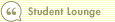Course 403: Introduction to Discounted Cash Flow
Discounting and Discount RatesIn this course1 Introduction 2 Estimating Future Cash Flow 3 Discounting and Discount Rates 4 Cost of Capital 5 Two Types of Capital, Two Costs 6 The Perpetuity Value 7 The Bottom LineOnce we project the cash flows we expect a company to generate in the future, we have to discount those future cash flows back to the present to account for the time value of money. After all, a dollar today is worth more than a dollar 10 years from now, because the dollar today can be invested to earn a return over the next 10 years.

Suppose it is possible to invest our money at a 5% annual rate of return. In that case, \$1 today will become \$1.05 one year from now. Two years from now, it will become \$1.1025 (\$1.05 x \$1.05). Three years from now, it will become \$1.053, or \$1.1576, and so on.

To find the present value of \$1 of future cash flow, divide that future cash flow by the appropriate multiplier from the above example. A cash flow of \$1 one year in the future is worth \$0.9524 (\$1/\$1.05) in the present. If we invest that \$0.9524 at 5%, in one year we'll have exactly \$1. A \$1 cash flow two years in the future is worth \$1/\$1.1025, or \$0.9070, in the present. The further into the future we go, the less a given cash flow is worth right now. Generalizing this concept, the following formula is quite important:

Present Value of Cash Flow in Year N =
CF at Year N / (1 + R)^N

CF = Cash Flow
R = Required Return (Discount Rate)
N = Number of Years in the Future

Let's go through a few more examples. Suppose we have a \$1,000 cash flow three years in the future with a 7% rate of return. The present value of that cash flow is:

\$1,000 / (1 + .07)^3 = \$816.30

The same cash flow five years in the future would be worth:

\$1,000 / (1 + .07)^5 = \$712.99

And finally, a \$1,000 cash flow five years from now, but this time with a 10% discount rate, would be worth:

\$1,000 / (1 + .10)^5 = \$620.92

As you can see from these examples, the further out a cash flow is, the less it is worth in today's dollars. Also, the higher the rate of return used to discount the future cash flow, the lower the present value.

Next: Cost of Capital >>Search Print Lesson  | FeedbackDel.icio.us |digg itLearn how to invest like a pro with Morningstar’s Investment Workbooks (John Wiley & Sons, 2004, 2005), available at online bookstores.© Copyright 2015 Morningstar, Inc. All rights reserved. Please read our Privacy Policy. If you have questions or comments please contact Morningstar.